Test: Fractions- 2

# Test: Fractions- 2

Test Description

## 10 Questions MCQ Test Mathematics for Class 5 | Test: Fractions- 2

Test: Fractions- 2 for Class 5 2023 is part of Mathematics for Class 5 preparation. The Test: Fractions- 2 questions and answers have been prepared according to the Class 5 exam syllabus.The Test: Fractions- 2 MCQs are made for Class 5 2023 Exam. Find important definitions, questions, notes, meanings, examples, exercises, MCQs and online tests for Test: Fractions- 2 below.
Solutions of Test: Fractions- 2 questions in English are available as part of our Mathematics for Class 5 for Class 5 & Test: Fractions- 2 solutions in Hindi for Mathematics for Class 5 course. Download more important topics, notes, lectures and mock test series for Class 5 Exam by signing up for free. Attempt Test: Fractions- 2 | 10 questions in 15 minutes | Mock test for Class 5 preparation | Free important questions MCQ to study Mathematics for Class 5 for Class 5 Exam | Download free PDF with solutions
 1 Crore+ students have signed up on EduRev. Have you?
Test: Fractions- 2 - Question 1

### What is the product obtained when a fraction is multiplied by its reciprocal?

Detailed Solution for Test: Fractions- 2 - Question 1

The product of a fraction and its reciprocal is 1.

Test: Fractions- 2 - Question 2

### What is the sum of the reciprocals of 9 and 2 / 9?

Detailed Solution for Test: Fractions- 2 - Question 2

Reciprocals of 9 and 2 / 9 are 1 / 9 and 9 / 2 respectively.
Their sum =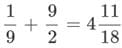Test: Fractions- 2 - Question 3

### Observe the number line given.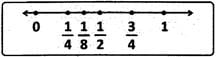Q. Which fraction represented on the number line is in a wrong place?

Detailed Solution for Test: Fractions- 2 - Question 3

1 / 8 < 1 / 4. So, it should lie between 0 and 1.

Test: Fractions- 2 - Question 4

Which fraction shows the part of the circle that is shaded?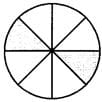Detailed Solution for Test: Fractions- 2 - Question 4

Test: Fractions- 2 - Question 5

How many tenths are there in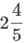?

Detailed Solution for Test: Fractions- 2 - Question 5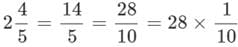Hence, there are 28 tenths inTest: Fractions- 2 - Question 6

Find the fraction greater than 7 / 8

Detailed Solution for Test: Fractions- 2 - Question 6

Convert all fractions to those with the same denominators and compare their numerators.
∴ The fraction greater than 7 / 8 is 11 / 12.

Test: Fractions- 2 - Question 7

Observe the given equation.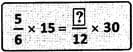Q. Identify the missing number in the box.

Detailed Solution for Test: Fractions- 2 - Question 7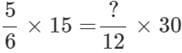On cancelling the factors common to numerator and denominator on both the sides,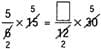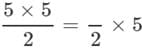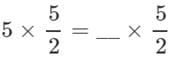Hence the missing number is 5.

Test: Fractions- 2 - Question 8

The sum of the numerator and the denominator of a fraction is 67. When 31 is added to the denominator, the fraction becomes 3 / 11. What was the original fraction?

Detailed Solution for Test: Fractions- 2 - Question 8

Numerator + Denominator = 67
After adding 31 to the denominator, the numerator is 3 units and denominator is 11 units.
3 + 11 = 14 units = 67 + 31 = 98
∴ 1 unit = 98 ÷ 14 = 7
3 units = 3 × 7 = 21 and 11 units = 11 × 7 = 77
New fraction = 21 / 77
Hence, the original fraction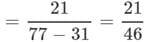Test: Fractions- 2 - Question 9

2 boys took a test. Pavan scored 4 / 5 of what Prateek had scored. If Pavan scored 76 marks, what was their total marks?

Detailed Solution for Test: Fractions- 2 - Question 9

Score of Pavan = 76 = 4 / 5 of score of Prateek 4 / 5 → 76,
5 / 5 → 76 × 5 / 4
= 19 × 5 = 95
∴ Their total marks = 76 + 95 = 171.

Test: Fractions- 2 - Question 10

Manish had Rs.45. He spent 3 / 5 of it on a birthday present. How much had he left?

Detailed Solution for Test: Fractions- 2 - Question 10

Fraction of the amount spent = 35
∴ Amount left =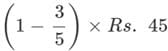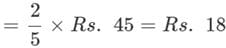## Mathematics for Class 5

12 videos|55 docs|35 tests
 Use Code STAYHOME200 and get INR 200 additional OFF Use Coupon Code
Information about Test: Fractions- 2 Page
In this test you can find the Exam questions for Test: Fractions- 2 solved & explained in the simplest way possible. Besides giving Questions and answers for Test: Fractions- 2, EduRev gives you an ample number of Online tests for practice

## Mathematics for Class 5

12 videos|55 docs|35 tests